# JP4124841B2 - Network analyzer, high frequency characteristic measurement apparatus, and error factor measurement method - Google Patents

Network analyzer, high frequency characteristic measurement apparatus, and error factor measurement method Download PDF

## Info

Publication number
JP4124841B2
JP4124841B2 JP19418897A JP19418897A JP4124841B2 JP 4124841 B2 JP4124841 B2 JP 4124841B2 JP 19418897 A JP19418897 A JP 19418897A JP 19418897 A JP19418897 A JP 19418897A JP 4124841 B2 JP4124841 B2 JP 4124841B2
Authority
JP
Japan
Prior art keywords
element
reflection coefficient
short
open
Prior art date
Legal status (The legal status is an assumption and is not a legal conclusion. Google has not performed a legal analysis and makes no representation as to the accuracy of the status listed.)
Expired - Fee Related
Application number
JP19418897A
Other languages
Japanese (ja)
Other versions
JPH1138054A (en
Inventor

Original Assignee

Priority date (The priority date is an assumption and is not a legal conclusion. Google has not performed a legal analysis and makes no representation as to the accuracy of the date listed.)
Filing date
Publication date
Application filed by 株式会社アドバンテスト filed Critical 株式会社アドバンテスト
Priority to JP19418897A priority Critical patent/JP4124841B2/en
Publication of JPH1138054A publication Critical patent/JPH1138054A/en
Application granted granted Critical
Publication of JP4124841B2 publication Critical patent/JP4124841B2/en
Anticipated expiration legal-status Critical
Application status is Expired - Fee Related legal-status Critical

## Images

•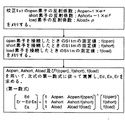•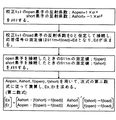•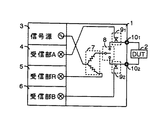•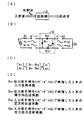•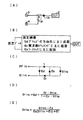•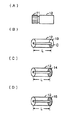## Description


BACKGROUND OF THE INVENTION
The present invention relates to a calibration method for a network analyzer.

[Prior art]
First, a general description of the network analyzer will be given. The network analyzer measures frequency characteristics of a high frequency among electrical quantities of circuit networks, electronic components, and electronic materials. This is a measuring device that generates a sine wave minute electric signal and applies it to a DUT (device under test), and measures and analyzes its reflection characteristic and transmission characteristic, that is, a response signal using S parameters. The response signal is generally a vector quantity having amplitude and phase information, and is a complex number. Therefore, a measuring device that analyzes the vector quantity of the amplitude and phase is also called a vector network analyzer.

Since the internal configuration of the network analyzer is well known, it is omitted, and the basic configuration with a built-in S parameter test set is shown in FIG. In the figure, 1 is a network analyzer with an S-parameter test set, 2 is a DUT, 3 is a signal source, and generally uses a swept oscillator. 4 is a receiving unit A, which receives a received signal with a mixer, converts it to a low frequency, performs analog / digital conversion (A / D), performs quadrature detection, and obtains a real value R and an imaginary value X as one complex number. Measured. Reference numeral 5 denotes a receiving unit R that measures a transmission signal from the signal source 3. Reference numeral 6 denotes a receiving unit B. These three receiving units are synchronized so as to detect a signal having a frequency output from the signal source 3.

Reference numeral 7 denotes a power splitter for separating the signal from the signal source 3. One signal is given to the DUT 2 through the RF switch 8, and the other signal is given to the receiving unit R5. The RF switch 8 is for outputting an output signal from the signal source 3 from the port 1 of the terminal 10 1 or from the port 2 of the terminal 10 2 . Reference numerals 9 1 and 9 2 denote bridges or directional couplers for extracting response signals from the terminal 10 1 or the terminal 10 2 . This S parameter test set built-in network analyzer 1 measures the S parameter of the DUT 2.

The S parameter will be briefly described with reference to FIG. When the measurement frequency becomes high and the measurement system can no longer handle the lumped constant, as shown in FIG. 4A, the incident wave, the reflected wave, and the transmitted wave are defined as variables as the parameters of the circuit network and measured. The defined network parameter is the S parameter. For example, as shown in FIG. 4B, it is assumed that the signal a1 is given from the signal source 3 to the port 1 of the DUT 2 and the port 2 is terminated with the characteristic impedance Zo. S11 at this time is defined as the ratio of incident wave a1 and reflected wave b1 at port 1, S11 = b1 / a1, and is called the reflection coefficient at port 1. S21 is defined as the ratio of the transmission wave b2 from port 1 to port 2 and the incident wave a1 of port 1, S21 = b2 / a1, and is called a transmission coefficient or transmission coefficient from port 1 to port 2. S22 and S21 are measured by applying the signal a2 from the port 2 and terminating the port 1 with the characteristic impedance Zo, and are defined as S22 = b2 / a2 and S12 = b1 / a2.

FIG. 4C represents this relational expression in a matrix. FIG. 4D illustrates the contents of the S parameter. The network analyzer 1 converts the S parameters thus obtained into various characteristics of the DUT 2 and displays them. For example, LOGMAG display that displays the amplitude in dB, PHASE display that displays the phase, DELAY display of the group delay time, SWR display of the standing wave ratio, SMITH display of the Smith chart, POLAR display of the polar chart, and the like.
By the way, when the reflection characteristic of the DUT 2 is to be measured by the network analyzer 1, the true value of the DUT 2 cannot be directly measured due to a measurement system error. Therefore, it is possible to correct the measured value by knowing the cause of this error and considering an appropriate model.

Next, measurement of a conventional network analyzer related to the present invention will be described below with reference to FIG. FIG. 5A shows a measurement system that measures the reflection characteristics of the DUT 2 with a network analyzer. A signal from the signal source 3 is given to the DUT 2 and the reflected wave is taken out by the bridge 9 and measured by the receiving unit A4.

FIG. 5B shows the measurement error factors in this case. That is, it is an error mainly caused by the directionality of the measurement system, frequency tracking, and source match. The directionality error means that the incident signal directed to DUT2 and the reflected signal from DUT2 must be separated by bridge 9, but the measured value S11m includes leakage from the forward direction, that is, a leakage signal. This is an error. The error due to frequency tracking is an error in the frequency response of the measurement system. The error due to source match means that when the impedance of the signal source side and the impedance of the measurement system system are not matched, the signal reflected by the DUT 2 is reflected again by the signal source 3 side and returned to the DUT 2 and re-reflected. . This is an error due to re-reflection.

Including these, the error model for measuring the reflection characteristics of one port is as shown in FIG. Here, S11m is a measured value, S11a is a true value, and Ed, Er, and Es are error factors. Although the explanation of this error model is omitted, it can be expressed by FIG. 5D when S11m is obtained by solving with a signal flow graph. If the true value S11a is obtained by deformation, it can be expressed in FIG. Here, since there are three unknowns, Ed, Er, and Es, these three unknowns can be obtained by using three standard devices with known characteristics.

That is, three states of open (release), short (short-circuit) and load (standard load Zo) are created, and the measured values f (short), f (open) and f (load) of S11m at each time are obtained. If it is recorded and calculated using the value, the true reflection coefficient S11a of DUT2 can be obtained. This is called calibration. That is, the calibration is to measure an error of the measurement system in advance and remove the influence by calculation.

There are calibration kits to create open, short and load conditions. An example is shown in FIG. FIG. 6A is an external view, 11 is a connector, and 12 is a main body. FIG. 6B is an open element, and the terminal 13 is open, but the stray capacitance C and the like exist, so the reflection coefficient Aopen is (1 × e j α) in consideration of phase correction. FIG. 6C shows a short element, the terminal 14 is short-circuited, and the reflection coefficient Ashort is (−1 × e j β) in consideration of phase correction. FIG. 6D assumes that the load element is a load element, the terminal 15 is terminated with a characteristic impedance Zo, and the reflection coefficient is zero. In general, the characteristic impedance Zo is often 50Ω and 75Ω.

A conventional calibration method is shown in FIG. First, the reflection coefficient Aopen = (1 × e j α) of the open element of the calibration kit and the reflection coefficient Ashort = (− 1 × e j β) of the short element are stored in the storage unit of the network analyzer 1.
Next, assuming that the load element is non-reflective, that is, the reflection coefficient = 0, the load element is connected to the terminal 10i, and the response signal is measured by the receiving unit A4. The measured value f (load) of S11m is obtained from the equation of FIG.
f (load) = S11m = Ed + {Er · 0 / (1−Es · 0)} = Ed
It becomes. That is, the measured value f (load) = Ed, and Ed is obtained.

Next, an open element is connected to the terminal 10i and its measured value f (open) is obtained and stored, and a short element is connected and its measured value f (short) is obtained and stored. here,
f (open) = f (load) + {Er · Aopen / (1−Es · Aopen)}
f (short) = f (load) + {Er · Ashort / (1−Es · Ashort)}
Therefore, the second equation is obtained by combining these two equations.
When the measurement values f (open) and f (short) are obtained, the reflection coefficients Aopen and Ashort are used to perform the calculation according to the second equation of Equation 2 to obtain Es and Er.

[Expression 2]

FIG. 2 shows a flowchart of the conventional calibration method described above. Memorize the reflection coefficients Aopen and Ashort of the open and short elements of the calibration kit. When the load element is assumed to have a reflection coefficient of 0 and connected to a terminal, a signal is applied from a signal source, and the response signal is measured, the measured value is S11m = f (load) = Ed, and Ed is obtained. Similarly, the measurement values f (open) and f (short) are measured and stored. Using these Aopen, Ashort, f (open), and f (short), calculation is performed according to the second formula to obtain Es and Er, and calibration is performed.

[Problems to be solved by the invention]
In the conventional calibration method, the load element of the calibration kit is assumed to have a reflection coefficient = 0. Therefore, he was eager to develop a non-reflective load element. In other words, the load element is only assumed to be ideal, and the reflection coefficient = 0, but in reality there is some reflection. Therefore, the non-ideal part of the load element becomes an error in calibration, causing a slight measurement error.

The present invention provides a new calibration method in which the reflection coefficient of the load element is not assumed to be 0, the reflection coefficient = ρ is a known value, and an error factor that does not cause a measurement error is obtained. With the goal.

[Means for Solving the Problems]
In order to achieve the above object, the present invention uses a calibration kit having a known reflection coefficient. The calibration kit includes three types of elements: open elements, short elements, and load elements. The reflection coefficient of each element, that is, the reflection coefficient Aopen of the open element, the reflection coefficient Ashort of the short element, and the reflection coefficient Aload of the load element are stored in the storage unit of the network analyzer.

Next, the open element is connected to the input / output terminal of the network analyzer, a signal is sent from the signal source, and the response signal is measured by the receiving unit A4 or the receiving unit B6. The measured value of S11m is f (open). The measured value of S11m measured with the short element connected to the terminal is defined as f (short). The measured value of S11m measured with the load element connected to the terminal is defined as f (load). The measured f (open), f (short) and f (load) are also stored in the storage unit of the network analyzer.

Next, using the above reflection coefficients Aopen, Ashort, Aload and the above measured values f (open), f (short), f (load), Ed, Es in the arithmetic unit according to the first formula of Equation 1. And Er are obtained to perform calibration.

[Equation 3]

DETAILED DESCRIPTION OF THE INVENTION
DESCRIPTION OF THE PREFERRED EMBODIMENTS Embodiments of the present invention will be described based on examples with reference to the drawings. FIG. 1 shows a flowchart of an embodiment of the calibration method of the present invention. The reflection coefficients of the three elements of the calibration kit are ideally Aopen = 1 for an open element, Ashort = −1 for a short element, and Aload = 0 for a load element. However, in reality it is not ideal and there are some errors. Therefore, the correction values α, β, and ρ are clarified by accurately measuring in advance, theoretically calculating, or purchasing a corrected one. The reflection coefficient is Aopen = (1 × e j α) for the open element, Ashort = (− 1 × e j β) for the short element, and Aload = ρ for the load element. This reflection coefficient is stored in the storage unit of the network analyzer.

Next, these three elements are alternately connected to the input / output terminal 10i of FIG. 5A to give a signal from the signal source 3, and the response signal is measured by the receiver A4. The measured value of S11m when the open element is connected is f (open), the measured value of S11m when the short element is connected is f (short), and the measured value of S11m when the load element is connected is f (load). ) Is stored in the storage unit.

By the way, the mathematical formula in FIG.
S11m = Ed + {Er · S11a / (1−Es · S11a)}
S11m = Ed + S11a (Er-Ed.Es) + S11a.S11m.Es. Here, substituting the measurement value described above for S11m and the reflection coefficient for S11a, the following three equations are obtained.
f (open) = Ed + Aopen (Er-Ed · Es) + Aopen · f (open) · Es
f (short) = Ed + Ashort (Er-Ed · Es) + Ashort · f (short) · Es
The first equation of Equation 1 is a matrix display of these three equations. These three equations can be calculated by the calculation unit to determine the unknown numbers of Ed, Es, and Er. That is, calibration can be performed without assuming Aload = 0.

【The invention's effect】
As described above in detail, according to the present invention, even if the reflection coefficient of the load element (also called load standard) is 0, that is, it is not ideal non-reflection, calibration is possible by calculation if the value is known. It was. Therefore, the load element can be easily manufactured, the cause of the measurement error is eliminated, and the utility value of the network analyzer is increased. The technical value of this invention is great.
[Brief description of the drawings]
FIG. 1 is a flowchart of an embodiment of a calibration method of the present invention.
FIG. 2 is a flowchart of a conventional calibration method.
FIG. 3 is a configuration diagram of an example of a network analyzer with a built-in S-parameter test set.
FIG. 4 is an explanatory diagram of S parameters. (A) is an explanatory diagram of incident waves, reflected waves, and transmitted waves, (B) is an explanatory diagram of individual S parameters, (C) is a relational expression of S parameters, and (D) is an explanation of individual S parameters. .
FIG. 5 is an explanatory diagram of 1-port reflection characteristic measurement. (A) is a configuration diagram, (B) is an explanatory diagram of measurement error, (C) is an error model diagram, (D) is a relational expression of measurement value S11m, and (E) is a relational expression of true value S11a.
FIG. 6 is an explanatory diagram of a calibration kit. (A) is an external view, (B) is an open element, (C) is a short element, and (D) is a load element.
[Explanation of symbols]
1 S-parameter test set built-in network analyzer 2 DUT (DUT)
3 Signal source 4 Receiver A
7 power splitter 8 RF switch 9i, 9 1 , 9 2 bridge or directional coupler 10i, 10 1 , 10 2 terminal 11 connector 12 body 13 open element terminal 14 short element terminal 15 load element terminal

## Claims (3)

1. A network analyzer ,
A known reflection coefficient recording unit for recording a known reflection coefficient of an open element that creates an open state, a short element that creates a short state, and a load element that creates a load state;
A measurement reflection coefficient recording unit for recording a measurement result of the reflection coefficient of the open element, the short element, and the load element by the network analyzer ;
The error factor Ed of the error mainly caused by the directionality of the network analyzer is the known reflection coefficient of the open element, the short element, and the load element, and the open element, the short element, and the load element. A directional error factor calculation unit that calculates based on the measurement result of the reflection coefficient;
An error factor Es of an error mainly caused by a source match is calculated using the known reflection coefficient of the open element, the short element, and the load element, and the reflection coefficient of the open element, the short element, and the load element. A source match error factor calculation unit that calculates based on the measurement result;
An error factor Er of an error mainly caused by frequency tracking is measured for the known reflection coefficient of the open element, the short element, and the load element, and the reflection coefficient of the open element, the short element, and the load element. A frequency tracking error factor calculation unit that calculates based on the result and the error factors Ed and Es;
With
Aopen for the known reflection coefficient of the open element,
The known reflection coefficient of the short element is Ashort,
The measurement result of the reflection coefficient of the open element is f (open),
The measurement result of the reflection coefficient of the short element is f (short),
The measurement result of the reflection coefficient of the load element is f (load),
If
The directional error factor calculation unit, the source match error factor calculation unit, and the frequency tracking error factor calculation unit perform calculation according to the first formula of Equation 1.
Network analyzer .
2. A high-frequency frequency characteristic measuring device ,
A known reflection coefficient recording unit for recording a known reflection coefficient of an open element that creates an open state, a short element that creates a short state, and a load element that creates a load state;
A measurement reflection coefficient recording unit for recording a measurement result of the reflection coefficient of the open element, the short element, and the load element by the high frequency characteristic measurement device ;
The error factor Ed of the error mainly caused by the directivity of the high-frequency frequency characteristic measuring device is the known reflection coefficient of the open element, the short element, and the load element, and the open element, the short element, and the load. A directional error factor calculation unit for calculating based on the measurement result of the reflection coefficient of the element;
An error factor Es of an error mainly caused by a source match is calculated using the known reflection coefficient of the open element, the short element, and the load element, and the reflection coefficient of the open element, the short element, and the load element. A source match error factor calculation unit that calculates based on the measurement result;
An error factor Er of an error mainly caused by frequency tracking is measured for the known reflection coefficient of the open element, the short element, and the load element, and the reflection coefficient of the open element, the short element, and the load element. A frequency tracking error factor calculation unit that calculates based on the result and the error factors Ed and Es;
With
Aopen for the known reflection coefficient of the open element,
The known reflection coefficient of the short element is Ashort,
The measurement result of the reflection coefficient of the open element is f (open),
The measurement result of the reflection coefficient of the short element is f (short),
The measurement result of the reflection coefficient of the load element is f (load),
If
The directional error factor calculation unit, the source match error factor calculation unit, and the frequency tracking error factor calculation unit perform calculations according to the first formula of Equation 2 ,
High frequency frequency characteristic measuring device .
3. An error factor measurement method for measuring an error factor in a high frequency characteristic measurement device ,
A known reflection coefficient recording step of recording a known reflection coefficient of an open element that creates an open state, a short element that creates a short state, and a load element that creates a load state;
A measurement reflection coefficient recording step for recording a measurement result of the reflection coefficient of the open element, the short element, and the load element by the high frequency characteristic measuring device ;
The error factor Ed of the error mainly caused by the directivity of the high-frequency frequency characteristic measuring device is the known reflection coefficient of the open element, the short element, and the load element, and the open element, the short element, and the load. A directional error factor calculation step for calculating based on the measurement result of the reflection coefficient of the element;
An error factor Es of an error mainly caused by a source match is calculated using the known reflection coefficient of the open element, the short element, and the load element, and the reflection coefficient of the open element, the short element, and the load element. Source match error factor calculation process that calculates based on the measurement result;
An error factor Er of an error mainly caused by frequency tracking is measured for the known reflection coefficient of the open element, the short element, and the load element, and the reflection coefficient of the open element, the short element, and the load element. A frequency tracking error factor calculation step to calculate based on the result and the error factors Ed and Es;
With
Aopen for the known reflection coefficient of the open element,
The known reflection coefficient of the short element is Ashort,
The measurement result of the reflection coefficient of the open element is f (open),
The measurement result of the reflection coefficient of the short element is f (short),
The measurement result of the reflection coefficient of the load element is f (load),
If
The directional error factor calculation step , the source match error factor calculation step, and the frequency tracking error factor calculation step perform calculations according to the first equation of Equation 3 .
Error factor measurement method.
JP19418897A 1997-07-18 1997-07-18 Network analyzer, high frequency characteristic measurement apparatus, and error factor measurement method Expired - Fee Related JP4124841B2 (en)

## Priority Applications (1)

Application Number Priority Date Filing Date Title
JP19418897A JP4124841B2 (en) 1997-07-18 1997-07-18 Network analyzer, high frequency characteristic measurement apparatus, and error factor measurement method

## Applications Claiming Priority (1)

Application Number Priority Date Filing Date Title
JP19418897A JP4124841B2 (en) 1997-07-18 1997-07-18 Network analyzer, high frequency characteristic measurement apparatus, and error factor measurement method

## Publications (2)

Publication Number Publication Date
JPH1138054A JPH1138054A (en) 1999-02-12
JP4124841B2 true JP4124841B2 (en) 2008-07-23

# Family

## Family Applications (1)

Application Number Title Priority Date Filing Date
JP19418897A Expired - Fee Related JP4124841B2 (en) 1997-07-18 1997-07-18 Network analyzer, high frequency characteristic measurement apparatus, and error factor measurement method

## Country Status (1)

JP (1) JP4124841B2 (en)

## Families Citing this family (15)

* Cited by examiner, † Cited by third party
Publication number Priority date Publication date Assignee Title
JP2001153904A (en) 1999-11-25 2001-06-08 Advantest Corp Network analyzer, network analyzing method and storage medium
JP4255017B2 (en) * 2002-04-17 2009-04-15 株式会社アドバンテスト Automatic calibrator, calibration method, program and recording medium
US7302351B2 (en) 2002-11-27 2007-11-27 Advantest Corporation Power supply device, method, program, recording medium, network analyzer, and spectrum analyzer
JP4506154B2 (en) * 2003-01-08 2010-07-21 凸版印刷株式会社 Characteristic impedance measuring method and measuring apparatus
JP4274462B2 (en) 2003-09-18 2009-06-10 株式会社アドバンテスト Error factor acquisition apparatus, method, program, and recording medium
JP5242881B2 (en) 2004-02-23 2013-07-24 ローデ ウント シュワルツ ゲーエムベーハー ウント コー カーゲー Network analyzer, network analysis method, program, and recording medium
JP4462979B2 (en) 2004-03-26 2010-05-12 株式会社アドバンテスト Network analyzer, transmission tracking measurement method, network analysis method, program, and recording medium
EP1752777A4 (en) * 2004-05-14 2009-06-17 Panasonic Corp Method and apparatus for measuring electric circuit parameter
DE112005001501T5 (en) * 2004-06-28 2007-05-16 Advantest Corp A measuring device, method, program, storage medium, network analyzer and semiconductor tester for measuring characteristics of a connector
KR100840923B1 (en) 2004-06-28 2008-06-24 가부시키가이샤 아드반테스트 Connecting element characteristic measurement device, method, program, recording medium, network analyzer, and semiconductor test device
TWI338784B (en) 2006-08-30 2011-03-11 Advantest Corp
JP4188396B2 (en) 2006-08-31 2008-11-26 株式会社アドバンテスト Error factor determination apparatus, method, program, recording medium, output correction apparatus including the apparatus, and reflection coefficient measurement apparatus
US7809216B2 (en) * 2007-03-16 2010-10-05 Photonic Systems, Inc. Bi-directional signal interface and apparatus using same
US7761253B2 (en) 2007-07-23 2010-07-20 Advantest Corporation Device, method, program, and recording medium for error factor measurement, and output measurement device and input measurement device provided with the device for error factor measurement
US7616007B2 (en) 2007-07-23 2009-11-10 Advantest Corporation Device, method, program, and recording medium for error factor measurement, and output correction device and reflection coefficient measurement device provided with the device for error factor measurement

## Also Published As

Publication number Publication date
JPH1138054A (en) 1999-02-12

## Similar Documents

Publication Publication Date Title
Basu et al. An SOLR calibration for accurate measurement of orthogonal on-wafer DUTs
Hoer A network analyzer incorporating two six-port reflectometers
CA2549698C (en) Signal measurement systems and methods
US6701265B2 (en) Calibration for vector network analyzer
Engen A (historical) review of the six-port measurement technique
US7107170B2 (en) Multiport network analyzer calibration employing reciprocity of a device
Engen et al. Application of an arbitrary 6-port junction to power-measurement problems
JP2005055438A (en) Improved direct path, reflective path, method and device of measurement of device to be tested using line measurement calibration
Rytting Network analyzer error models and calibration methods
DE102004020037B4 (en) Calibration method for performing multi-port measurements on semiconductor wafers
Ferrero et al. An improved calibration technique for on-wafer large-signal transistor characterization
US4962359A (en) Dual directional bridge and balun used as reflectometer test set
JP2698640B2 (en) Calibration method and an optical component measurement system
Engen The six-port reflectometer: An alternative network analyzer
US6665628B2 (en) Methods for embedding and de-embedding balanced networks
DE19828682B4 (en) Automatic calibration of a network analyzer
US4853613A (en) Calibration method for apparatus evaluating microwave/millimeter wave circuits
KR100456493B1 (en) Multi-port device analysis apparatus and method and calibration method thereof
US7254511B2 (en) Method and apparatus for calibrating a frequency domain reflectometer
US4041395A (en) Transmitter performance monitor and antenna matching system
US6882160B2 (en) Methods and computer program products for full N-port vector network analyzer calibrations
US7786737B2 (en) Modeling and calibrating a three-port time-domain reflectometry system
US20050156585A1 (en) Reduced complexity transmission line and waveguide fault tester
US6853198B2 (en) Method and apparatus for performing multiport through-reflect-line calibration and measurement
US6188968B1 (en) Removing effects of adapters present during vector network analyzer calibration

## Legal Events

Date Code Title Description
A621 Written request for application examination

Free format text: JAPANESE INTERMEDIATE CODE: A621

Effective date: 20040304

A977 Report on retrieval

Free format text: JAPANESE INTERMEDIATE CODE: A971007

Effective date: 20060403

A131 Notification of reasons for refusal

Free format text: JAPANESE INTERMEDIATE CODE: A131

Effective date: 20070320

RD02 Notification of acceptance of power of attorney

Free format text: JAPANESE INTERMEDIATE CODE: A7422

Effective date: 20070517

A521 Written amendment

Free format text: JAPANESE INTERMEDIATE CODE: A523

Effective date: 20070517

A131 Notification of reasons for refusal

Free format text: JAPANESE INTERMEDIATE CODE: A131

Effective date: 20080227

A521 Written amendment

Free format text: JAPANESE INTERMEDIATE CODE: A523

Effective date: 20080415

TRDD Decision of grant or rejection written
A01 Written decision to grant a patent or to grant a registration (utility model)

Free format text: JAPANESE INTERMEDIATE CODE: A01

Effective date: 20080430

A01 Written decision to grant a patent or to grant a registration (utility model)

Free format text: JAPANESE INTERMEDIATE CODE: A01

A61 First payment of annual fees (during grant procedure)

Free format text: JAPANESE INTERMEDIATE CODE: A61

Effective date: 20080507

R150 Certificate of patent or registration of utility model

Free format text: JAPANESE INTERMEDIATE CODE: R150

FPAY Renewal fee payment (event date is renewal date of database)

Free format text: PAYMENT UNTIL: 20110516

Year of fee payment: 3

FPAY Renewal fee payment (event date is renewal date of database)

Free format text: PAYMENT UNTIL: 20110516

Year of fee payment: 3

FPAY Renewal fee payment (event date is renewal date of database)

Free format text: PAYMENT UNTIL: 20120516

Year of fee payment: 4

FPAY Renewal fee payment (event date is renewal date of database)

Free format text: PAYMENT UNTIL: 20130516

Year of fee payment: 5

FPAY Renewal fee payment (event date is renewal date of database)

Free format text: PAYMENT UNTIL: 20130516

Year of fee payment: 5

FPAY Renewal fee payment (event date is renewal date of database)

Free format text: PAYMENT UNTIL: 20130516

Year of fee payment: 5

FPAY Renewal fee payment (event date is renewal date of database)

Free format text: PAYMENT UNTIL: 20140516

Year of fee payment: 6

LAPS Cancellation because of no payment of annual fees